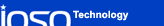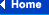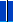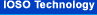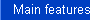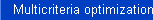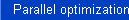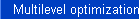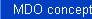### IOSO algorithms equally well handle optimization problems with various types of objective function

We conducted extensive tests of estimating IOSO Technology algorithms efficiency for the following types of objective functions:

• smooth;
• stochastic;
• non-differentiable;
• dependent on mixed variables (continuous and discrete);
• with the region in the design space where the objective function can not be evaluated.

The figure below shows the results for the test functions collected by Eric Sandgren (Sandgren, Eric. “The Utility of Nonlinear Programming Algorithms”, a thesis submitted for the Degree of Doctor of Philosophy, Purdue Univercity, 1977). Each of these functions was considered initially in the original formulation and then was modified to model the objective functions of various types.

The obtained results confirm that IOSO Technology algorithms are practically independent of the objective function types and can be successfully applied in various science and technology fields.

##### Comparative score: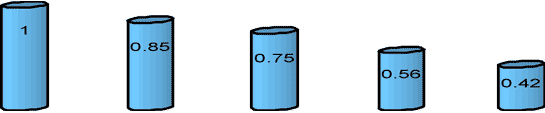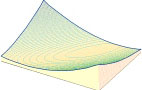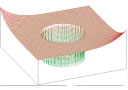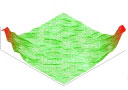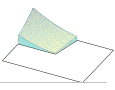smooth; non-differentiable; stochastic; dependent on mixed variables (continuous and discrete) with the non-calculation region